# Optimizing Magnetic Core Gapping for Low-Loss Sine-Wave Inductors

By Kenneth Pagenkopf, Principal Engineer – Acme Electric

Improving Energy Efficiency – Redesigning inductors to reduce their losses is a requirement for high-efficiency power conversion. Starting with a basic understanding of the construction and applications of these components and moving to a critical analysis of the mechanisms and theory behind the magnetic field fringing that occurs will lead to a methodology of predicting gap sizes and inductance that minimizes losses and improves performance. A few simple formulas can help find design limits and a more comprehensive approach can help the designer establish optimal positions, quantities and sizes for gaps within inductor cores. There are additional benefits that can be realized from this approach, which allow for smaller size and lower costs. A broad-thinking design approach based in solid magnetic equations can help optimize magnetic core gapping for low-loss sine-wave inductors.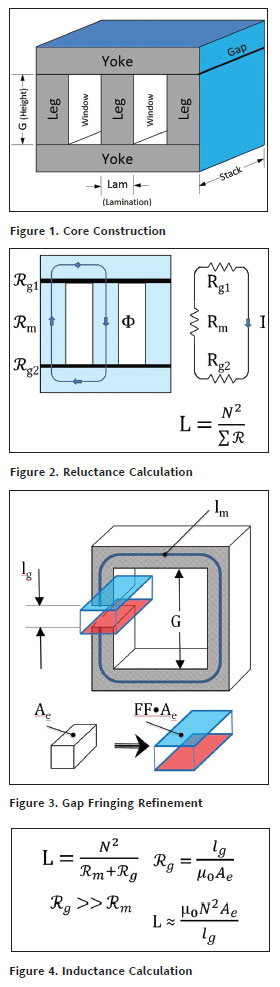Inductor Applications – Three-phase sine-wave inductors are used in many types of equipment that convert energy using pulse-width modulation (PWM) techniques. These include motor drives, inverters for alternative energy and regenerative systems. The PWM developed needs to be made into a clean, smooth, sine-wave for power drive and energy supply applications. Even though there are electronic means of accomplishing this, it is still primarily done by passive filtering with inductors and capacitors. Traditional construction of the three-phase inductors can be done by combining three single-phase components or by the use of a single three-phase inductor. Then, these can be constructed by using powdered cores, wound amorphous cores or laminated silicon steel cores. This discussion will be directed toward the construction of three-phase laminated silicon steel core sine-wave inductors.

Steel Core Construction – Standard steel-core construction involves three legs and two yokes of laminated material having a consistent width (lamination) and depth (stack) as shown in Figure 1. The structure is clamped together and is usually glued for audible noise reduction. Each leg of the core is surrounded by a wound coil and there is at least one gap in each of the core legs. The gaps serve to allow the adjustment of inductance and control flux density in design. The quantity, size and placement of these gaps can have a large impact on the power losses of the assembly and the inductance balance between legs. Understanding how they work and how to predict their effects is the key to optimizing efficiency in inductor design.

Gapping Theory – Air gaps affect inductance through reluctance and flux. When magnetic flux goes around a magnetic circuit, it is much like current in a resistive circuit and the inductance of the inductor is most simply calculated by the circuit shown in Figure 2. With multiple gaps, the reluctance is summed linearly. Yet, the magnetic field in an air gap is not as tightly constrained to the core’s cross-sectional area as it is in the core. The field fringes outward around the gap and the amount of fringing is affected by what magnetic structures and fields are around it. When fringing occurs outside the coils, the core structure bends it and when within the coils, the coils constrain it. Much like skin effect in conductors, in operating inductors, the coils create opposing forces that push back on the fringing and reduce its effects. A simple way to see the effects of fringing is to envision it creating a larger effective cross-sectional area (Ae) as it goes through the gap as shown in Figure 3. This will make the inductance look larger. Basic formulas shown in Figure 4 show that the reluctance of the core can usually be neglected. This is because the air gap reluctance is significantly larger when air gaps and steel permeability are reasonably large. It is also seen that inductance is directly proportional to Ae.

Inductance Prediction – Considering that fringing affects the perception of the size of Ae, it is natural to see that inductance is affected by fringing. The common way to show this is by adding a fringing factor (FF) to the basic inductor equation as shown in Figure 5. This FF has been a subject of several investigations and the most prominent of these is from Colonel William T. McLyman . He demonstrated the equation shown in Figure 5, which comes from Reuben Lee . This works well for single small gaps on square stack construction, but can have significant errors in large single and multiple gap situations . Keith Williams  went further by attempting to calculate FF for each gap, but their summation is unclear for multiple gaps and there is still a non-square stack error. A new gapping formula is hereby proposed in Figure 6. It includes two new constants, Ks (the rectangular stack adjustment) and Kf, a fringing constant based on calculations from a newly proposed “Fundamental Gap Theory”, as well as a consideration of the effect of large gaps on the overall window height.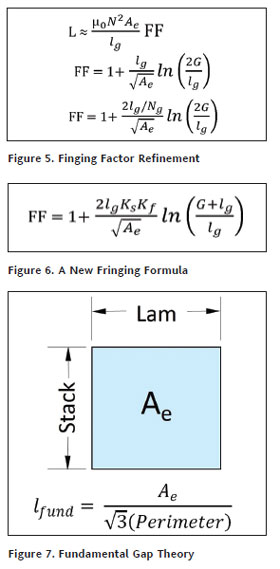Fundamental Gap Theory – The common FF formulas continue to show increasing fringing with increasing gap size and go indeterminate if gaps size is infinite. This is essentially the same as having no top yoke. Because of this, a designer may think that a fixed single top gap can produce any inductance. However, this is not the case. There is a minimum inductance that can be developed in any single top gap structure and this can be predicted from the Fundamental Gap. The “Fundamental Gap” is the equivalent distributed gap that matches the single infinite top gap. This can be predicted by knowing only the lamination and stack size of a three-phase steel core structure as shown in Figure 7. It assumes that the coils are reasonably centered on the core leg, sized to the core leg, and in a window that is closely matched to the lamination size. As gaps become completely distributed, the size becomes very small  and the inductance fringing factor will approach unity. Using an approximate multiplier of 1.17 and an intermediate form of the FF formula, which includes Ks, the “Fundamental Inductance” can be calculated as shown in Figure 8. This represents the minimum possible inductance that can be achieved by a single infinite top gap and puts a limit on the inductance prediction. Comparison of the formula to actual test and simulated* results for square and rectangular cores ranging from 0.625-inch to 5-inch lamination showed a correlation within ±10 percent over 80 percent of the time.

Applying the Theory – Knowing the effect of the coils on fringing, a means of differentiating the fringing calculation for each gap location relative to the coil is necessary. Analyzing actual test and simulated* results helped derive an empirical formula for each position as shown in Figure 9. The largest Kf comes from the top gap, which is not shadowed by the coil and is calculated directly from the Fundamental Gap. It then proceeds by a cube root progression to the center gap, which is entirely encompassed by the coil. The completely developed formula for predicting inductance is shown in Figure 10. It includes the basic turns and reluctance summing, the Rm<<Rg assumption, the Ks calculation for rectangular cores, the new Kf calculation, and a compensation for the number of gaps. This was applied to a number of gap combinations in 5 different core sizes and was found to match actual and simulated* results to within ±10 percent over 80 percent of the time for multiple gap cores. As gaps exceeded the Fundamental Gap in single gap configurations, the error increased, but was still much better than predictions from historical formulas.  The use of the Fundamental Gap Theory significantly improves the prediction of inductance in three-phase laminated steel core structures.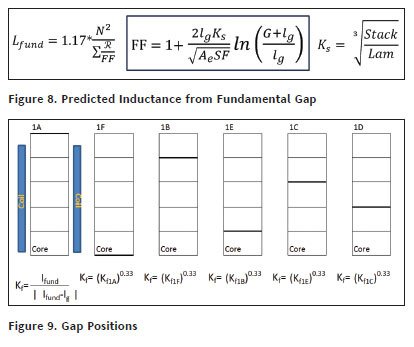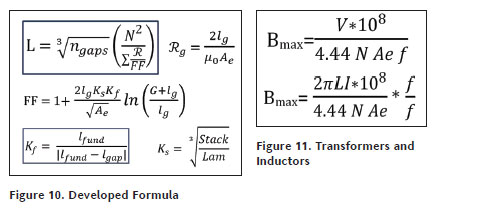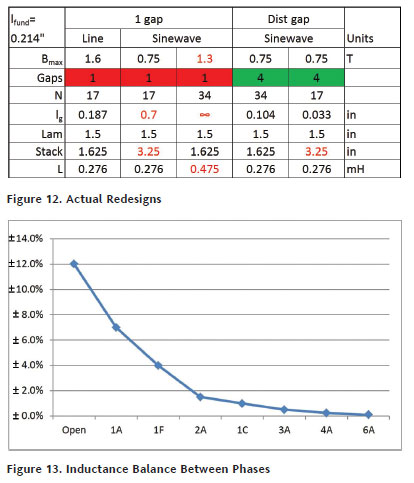Flux Density Management – With an understanding of the effect of fringing on inductance, the effect on flux density should also be reviewed. Starting with a simplified form of Faraday’s law as shown in Figure 11, which is regularly used by transformer designers, and incorporating Ohm’s law, allows inductance to appear directly in the formula. When fringing increases inductance, the flux density increases, so distributed gapping not only preserves inductance, but also flux density. In designing sine-wave inductors, it is necessary to reduce flux density to control steel core losses at high frequency. It can be seen that the approach would involve increasing turns or increasing Ae if the current and inductance were fixed. Yet, in a traditionally single-gapped line reactor, the top gap may already be close to its maximum. Looking at the design example in Figure 12, one can see the drawbacks of the single-gap approach. If Ae is increased and turns are maintained, the gap increases to a very impractical 0.7 inches. Then, if the turns are increased and Ae kept constant, the gap goes infinite and the desired inductance cannot even be achieved. Distributing the gap allows the added turns to be accommodated due to the decreased FF. Gaps remain reasonable and flux density is maintained. If Ae is increased and turns are constant, then, an even smaller gap can be used. Reduced fringing can be utilized to maintain a minimum size or constant turns when it is necessary to reduce flux density for sine-wave inductor design.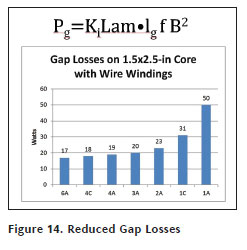Benefits of Distributed Gapping – Not only can size or turns be maintained with reduced flux density, there are other major benefits to using distributed gapping. The first of these is improved inductance balance between coils. Actual and simulated* data in Figure 13 shows that as gaps become smaller and moved to the center of the coil, imbalance can be reduced from ±8 percent in a single top gap to better than ±1 percent in a multi-gap configuration. Not surprisingly, smaller gaps result in smaller gap losses. From the formula in Figure 14, the reduction in losses from the gap length is linear, but when reduced gaps reduce fringing into the coils, the results can be much greater. In the example lab-tested design with wire windings**, gap losses were reduced 3:1 from 50 W to 17 W. Adding the effect of fringing on foil windings, the loss differences can be more dramatic. In one extreme example, gap losses were reduced from over 1,000 W to only 34 W. In wire windings, gaps should be well distributed with as many gaps as practical, but in foil windings, gaps should be distributed well, but to the center, not the edges. These final effects reinforce why distributed gapping can be so important to efficient sine-wave inductor design.

Optimized Core Gapping – Understanding gap fringing can indeed lead to optimized sine-wave inductor designs. The Fundamental Gap Theory reveals the limitations of single top gap designs and shows where distributed gaps are necessary. Then, as existing inductance prediction for multiple gaps is lacking, a formula based on the Fundamental Gap is provided that obtains good accuracy for most situations. It includes scalars for gap positions and quantities as well as rectangular core accommodation. With this, the designer can choose the desired gap distribution and choose the correct turns and cross-sectional area needed to maintain size and low loss performance. Then, the additional benefits of improved inductance imbalance, and lower gap and fringing losses can be realized. The optimal distributing and positioning of inexpensive laminated steel core gaps for three-phase sine-wave inductors can help achieve the small size, high energy efficiency and low cost necessary for today’s power conversion applications.

Footnotes:
* ANSYS Maxwell 3D FEA program was used to predict inductances and was confirmed by lab assembly and test.
** JFE Steel core materials aided in the separation of gap losses from core losses in measurements due to their low loss.

References:
 McLyman, Colonel William T. “Transformer and Inductor Design Handbook.” 3rd ed. New York: Marcel Dekker, 2004. Chapter 10. Print.
 “Flux Fringing” and “Distributed Air Gap,” (www.encyclopedia-magnetics.com)
 Williams, Keith L. “Practical Inductor Design.” EMCW Expo, Milwaukee, WI: May 9, 2013
 “Inductor and Flyback Transformer Design,” Section 5. (www. ti.com)
 Lee, Reuben “Electronic Transformers and Circuits” 2nd ed. New York: John Wiley, 1955. page 102About the Author
Kenneth Pagenkopf is an experienced designer of magnetic components used in passive filters for all spectra of unwanted noise.  Starting his career in SMPS power supplies, he observed first-hand their sources of generation and has spent the rest of his career trying to minimize them.  Whether the issue is 60Hz harmonics, fundamental switching frequencies, or EMI from switching transients, he has designed magnetics to mitigate them by working in a variety of materials which includes alloy powders, ferrites, amorphous and silicon steel.  He has a BSEE from Milwaukee School of Engineering, an MSPDD from Northwestern University and has been published previously in the field of EMI control.